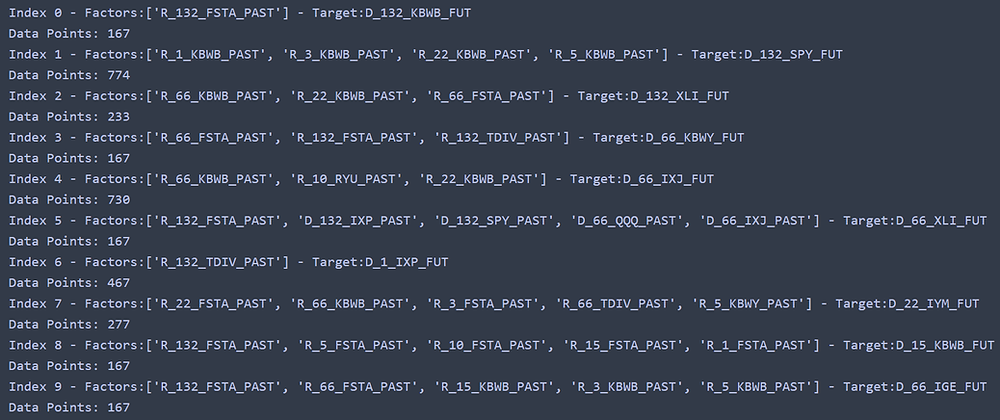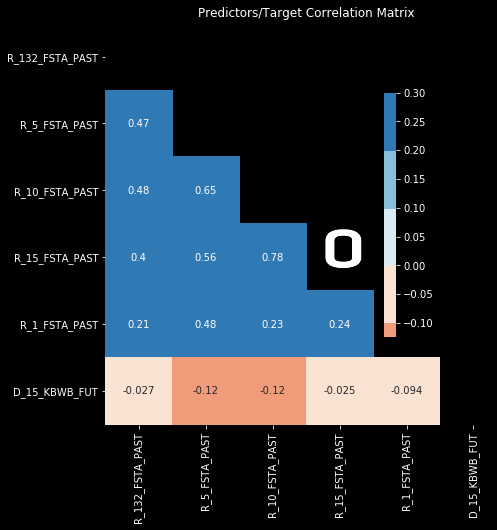Search

# Pattern Recognition in Sectorial ETFs: Past and Future with Predictive Power Score

The analysis we have performed, so far, for the price and returns relationships among sectorial ETFs has not been very conclusive. We have seen the current price and returns correlations and the future state correlations; these are generally high with very few exceptions and "stand-outs" for the period we are analyzing (2010 to 2020). We have not been able to properly observe strong negative correlations in this period but for a very few sector/market pairs and for very specific timeframes. As correlation seems not to be a promising evaluation tool to judge the usability of sector indexes to beat the market we will have to use alternative tools. We will evaluate the sectorial ETF information for its predictive power score this time.

Predictive Power Score (PPS) is a metric tool developed by 8080labs, the code and theoretical explanation can be found here. The PPS score will try to fit our data to a suitable decision tree (regression tree or classification tree) and generate a normalized score that gives us a view on how well a single variable explains or predicts one target variable. The model originally uses k folds to cross validate predictions and this may not be suitable to predict time series values, we have made a very quick modification to the PPS code to momentarily solve this issue. The modified code, with the very minor modification can be found here. We will try to formalize the time series split as an option for PPS instead of a hard change in the cross validation method.

For this predictive power analysis we will use the sectorial ETFs from previous analysis and enable the possible extension of the model to more traditional sector ETFs in the "X" series:

```self = QuantBook()
instruments = ['QQQ', 'SPY']

sector_ETF = ['IYM', 'XHB', 'FSTA', 'TPYP', 'KBWB', 'IXJ', 'XLI', 'KBWY', 'TDIV', 'IXP', 'RYU', 'IGE']
# sector_ETF = ['XLK', 'XLY', 'XLC', 'XLB', 'XLV', 'XLP', 'XLI', 'XLU', 'XLF', 'XLE', 'IGE']

start =  datetime(2005, 1, 1)
end = datetime(2015, 1, 1)

ETF_symbols = {etf: str(self.AddEquity(etf).Symbol.ID) for etf in sector_ETF}
pred_symbols = {symbol : str(self.AddEquity(symbol).Symbol.ID) for symbol in instruments}
all_symbols ={**ETF_symbols, **pred_symbols}

history = self.History(self.Securities.Keys, start, end,
Resolution.Daily,

As usual, the closing price can be extracted from the data that Quantconnect history call returns:

```price_history = history['close'].unstack(level=0)
inv_symbols = dict(map(reversed,all_symbols.items()))
price_history.columns = [inv_symbols[col] for col in price_history.columns]
price_history = pd.DataFrame(price_history)

In this extended 2005 to 2015 period that we are requesting this time some of the ETFs did not exist. There are multiple NaN values for prices in the history table that we are not going to clean for the time being, we will let our statistical tools know that these type of values have to be ignored.With Pandas shifting and pct_change() functions we can obtain the values for prices, returns and direction of the returns for multiple futures and pasts:

```time_frames = [1,3,5,10,15,22,66,132]
# P for price, R for returns, D for direction of the price change:
for column in price_history.columns:
for frame in time_frames:
price_history['P_'+str(frame)+"_"+column+'_FUT'] =  price_history[column].shift(-frame)
price_history['R_'+str(frame)+"_"+column+'_FUT'] = price_history[column].pct_change(-frame)
price_history['D_'+str(frame)+"_"+column+'_FUT'] = price_history[column].pct_change(-frame) > 0
price_history['R_'+str(frame)+"_"+column+'_PAST'] = price_history[column].pct_change(frame)
price_history['D_'+str(frame)+"_"+column+'_PAST'] = price_history[column].pct_change(frame) > 0```

We could at this stage normalize and winsorize the values to generate a more statistically "manageable" prices and returns series but we will not. We will prevent data leakage as the PPS module is designed to take in raw data and it does not have statistical transformations integrated into the score calculator. If we standardize and winsorize our values at this stage information of future prices would be used to obtain the predictive power score, masking the results a little bit.

For additional simplicity in data handling we will record the column names in lists that will allow us to access the cross sections for prices, returns, or direction:

```# Recording column names for simplicity of slicing:
cols = price_history.columns
price_cols = [col for col in cols if 'P_' in col]
returns_cols = [col for col in cols if 'R_' in col]
direction_cols = [col for col in cols if 'D_' in col]```

Now we can loop over all the columns containing the "future" and find the predictive power score for each of the "past" values. It takes a while, using tqdm.notebook to display the progress of the analysis will provide peace of mind. In this case 312 PPS calculations will take 20-30 minutes, depending on the machine:

```from tqdm.notebook import tqdm
import warnings
warnings.filterwarnings("ignore")

all_predictors = pd.DataFrame(columns=['x','y','ppscore'])
future_cols = [col for col in price_history.columns if 'FUT' in col]
past_cols = [col for col in price_history.columns if 'PAST' in col]

for column in tqdm(future_cols):
predictors_df = predictors(price_history[past_cols + [column]], y=column)
all_predictors = all_predictors.append(predictors_df[['x','y','ppscore']])```

The result is a dataframe with the predictive score for each of the future time frames for each of the ETFs when predicted by all of the pasts for all the ETFs. The prediction is one-to-one and does not provide any feature importance at this point. We can sort this dataframe by predictive power score to check what type of pairs are concentrated in the most powerful predictions:

```sorted_predictors = all_predictors.sort_values('ppscore', ascending=False).reset_index(drop=True)According to the PPS rank, past 132 day returns on FSTA (consumer stables) can predict the future price of KBWB (banks) 132 days into the future. The directionality of TDVI returns over 132 days (a categorical True/False series for dividend paying technology companies) can predict the prices of KBWY (real estate) in the short term (1 to 22 days ahead). These results are suspicious as we are saying, basically, that a lagging discrete signal is able to predict accurately a price value in a series of futures. Take into account that directionality is the signal in which more information is destroyed, after differentiating for the returns (operation that causes a loss of information) we are removing also the magnitude.

For these "top targets", targets with factors that have very high PPS, we can inspect the results of the score calculator. This reports the baseline scores, model scores and normalized score:

```power_leader = sorted_predictors.iloc['y']Let us continue by testing the most easily predictable variables, a simple classification problem and then, if we find out of sample predictive power, we can extend the work to returns and prices, which will require more "complex" regression models.

Determining which one is the most predictable variable is not a trivial task, as we have almost 65,000 pairs with predictive powers ranging from 0.65 to 0 (of a maximum possible of 1, a perfect prediction). If we take the sum of the total predictive powers, or the mean, we may be selecting a value composed of multiple weak signals. If we take the top value we may be discarding mid-table clusters of predicting variables that, when aggregated, provide the best possible prediction. We will use a hybrid path for this first trial, we will trim the standardized prediction scores using a given cut off standard deviation (3 for example) and obtain the average predictive power of these elements. We will also add a filter for the type of prediction as it will be easier for the models to predict initially the direction of the returns, it is also easier to implement in an eventual back-test. The 'D_' string will filter the best possible targets and predictors for the future direction of the returns:

```from scipy.stats import zscore
sorted_predictors['std_ppscore'] = sorted_predictors[['ppscore']].apply(zscore)
cut_off_devs = 3
pred_type = 'D_'
good_predictors = sorted_predictors[sorted_predictors['std_ppscore'] > cut_off_devs]
good_predictors = good_predictors[ good_predictors['y'].str.contains(pred_type) ].reset_index(drop=True)
good_targets = good_predictors.groupby('y')[['std_ppscore']].mean()
good_targets.sort_values('std_ppscore', ascending=False, inplace=True)The future direction of KBWB 132 days in the future carries the most predictive power using this method. It is important to check that, due to the differences in inception date, the available data for "these top targets" is sufficient to perform further analysis. To accomplish this we can iterate over the first few top targets and check how much data is available for train and test data sets according to both target and predictors history:

```N_top = 10
for i in range(N_top):
best_target = good_targets.iloc[i].name
best_predictors = list(good_predictors[good_predictors['y']==best_target]['x'])[:5]
print('Index {} - Factors:{} - Target:{}'.format(i,best_predictors, best_target))
data_qty = len(price_history[best_predictors +[best_target]].dropna())
print('Data Points: {}'.format(data_qty))```

This yields the top 10 targets, predictors and available data points for the analysis.It seems that the data for index 1 has the most data available, 774 points is more than 3 full years of data that we can use. It is also a multi-variable calculation, so that our results cannot be compared to single variable PPS results easily. As most of the predictions are in the top spots are very long term and our test data is relatively small in comparison we may have a single classification (False always for example) for the set, so we will spot check our model with either indexes 6 or 7, offering at the same time high predictive power, decent amount of data points and short term prediction:

```from sklearn.ensemble import RandomForestClassifier as rfc
from sklearn.metrics import f1_score
# In case we want to try to predict returns and/or price:
from sklearn.ensemble import RandomForestRegressor as rfr

INDEX = 7
best_target = good_targets.iloc[INDEX].name
best_predictors = list(good_predictors[good_predictors['y']==best_target]['x'])
display(predictors(price_history[best_predictors +[best_target]], y=best_target))
data = price_history[best_predictors +[best_target]].dropna()

total_samples = len(data)
n_train = int(total_samples*0.8)
train = data[:n_train]
test = data[n_train:]

X_train = train[train.columns[:-1]]
y_train = train[train.columns[-1]]
X_test = test[test.columns[:-1]]
y_test = test[test.columns[-1]]

clf = rfc(max_depth=10, random_state=0)
clf.fit(X_train, y_train);

print("Spot Model Score: {:.2f}".format(f1_score(y_test, clf.predict(X_test), average='weighted')))```

The spot random forest weighted f1 score is 0.83, in line with the 0.60 reported by the PPS model. The confusion matrix for this results can be obtained with:

```from sklearn.metrics import confusion_matrix as cm
plot_cm(cm(y_test, clf.predict(X_test)), title = 'Spot Model Confusion Matrix')```For smaller data sets, index 3 of our top performers, for example, the confusion matrix yields better results, it is calculated using very few test cases and even if we would like to believe this out of sample performance we have to be cautious, as there may be single predictable labels and the reported 100% score is plainly this, with no value at all:PPS seems to be working for this small sample, single target. We can further the analysis by checking now different classification models beyond tree models and work with a certain confidence that there is in fact a minimum amount of predictive power in our data. Predictive Power Score, seems, so far, capable of improving correlation and covariance analysis by providing another metric for future predictions.

Still we have not answered the original question: how is this predictive power related to correlation (or covariance)? and can we use all of these metrics to obtain better predictive models? This is a comparison for the future correlation of the values with the highest predictive powers:

```N_top = 10
for i in range(N_top):
best_target = good_targets.iloc[i].name
best_predictors = list(good_predictors[good_predictors['y'] == best_target]['x'])[:5]
df = price_history[best_predictors + [best_target]].dropna()

plot_corr_hm(df, title='Predictors/Target Correlation Matrix', annot=True)```

Among the correlation maps we obtain, in general, relatively low values for the target. For the matrix with the most factors in the top ten predictors, as an example:These results are but a curiosity as long as we cannot relate predictive power scores and correlation or covariance to obtain the best possible predictive factors. We can at least use the predictive groups to try and generate a trading model that uses the variables obtained from PPS analysis to enter the more "predictable" positions in this small sample ETF universe we have.

Remember that information in ostirion.net does not constitute financial advice, we do not hold positions in any of the companies or assets that we mention in our posts at the time of posting. If you are in need of algorithmic model development, deployment, verification or validation do not hesitate and contact us. We will be also glad to help you with your predictive machine learning or artificial intelligence challenges.

Here is the research code used in this post series in its unfinished state: